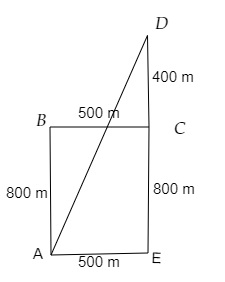# A man starts walking from point $A$ and reaches point $\mathrm{D}$. From $\mathrm{A}$ he walks towards north for $800 \mathrm{~m}$ to reach point $\mathrm{B}$. From $\mathrm{B}$ he walks towards east for $500 \mathrm{~m}$ to reach point C. From $\mathrm{C}$ he again walks towards north for $400 \mathrm{~m}$ to reach point $D$. Find the direct distance between points $\mathrm{A}$ and $\mathrm{D}$.

Given:

A man starts walking from point $A$ and reaches point $\mathrm{D}$. From $\mathrm{A}$ he walks towards north for $800 \mathrm{~m}$ to reach point $\mathrm{B}$. From $\mathrm{B}$ he walks towards east for $500 \mathrm{~m}$ to reach point C. From $\mathrm{C}$ he again walks towards north for $400 \mathrm{~m}$ to reach point $D$.

To do:

We have to find the direct distance between points $\mathrm{A}$ and $\mathrm{D}$.

Solution:From the figure,

The direct distance between points $\mathrm{A}$ and $\mathrm{D} =$ Hypotenuse of triangle AED

By Pythagoras theorem,

$AD^2=AE^2+DE^2$

$AD^2=(500)^2+(800+400)^2$

$=250000+1440000$

$=1690000$

$=(1300)^2$

$AD=1300\ m$

The direct distance between points $\mathrm{A}$ and $\mathrm{D}$ is 1300 m.

Updated on: 10-Oct-2022

24 Views